# integralFilter

Filter using integral image

## Syntax

```J = integralFilter(intI,H) ```

## Description

`J = integralFilter(intI,H)` filters an image, given its integral image, `intI`, and filter object, `H`. The `integralKernel` function returns the filter object used for the input to the `integralFilter`.

This function uses integral images for filtering an image with box filters. You can obtain the integral image, `intI`, by calling the `integralImage` function. The filter size does not affect the speed of the filtering operation. Thus, the `integralFilter` function is ideally suited to use for fast analysis of images at different scales, as demonstrated by the Viola-Jones algorithm .

## Tips

Because the `integralFilter` function uses correlation for filtering, the filter is not rotated before computing the result.

## Input Arguments

 `intI` Integral image. You can obtain the integral image, `intI`, by calling the `integralImage` function. The class for this value can be `double` or `single`. `H` Filter object. You can obtain the filter object, `H`, by calling the `integralKernel` function.

## Output Arguments

 `J` Filtered image. The filtered image, `J`, returns only the parts of correlation that are computed without padding. This results in `size`(`J`) = `size`(`intI`) – `H.Size` for an upright filter, and `size`(`J`) = `size`(`intI`) – `H.Size` – [0 1] for a rotated filter. This function uses correlation for filtering.

## Examples

collapse all

Read and display the input image.

``` I = imread('pout.tif'); imshow(I);```Compute the integral image.

` intImage = integralImage(I);`

Apply a 7-by-7 average filter.

``` avgH = integralKernel([1 1 7 7], 1/49); J = integralFilter(intImage, avgH);```

Cast the result back to the same class as the input image.

``` J = uint8(J); figure imshow(J);```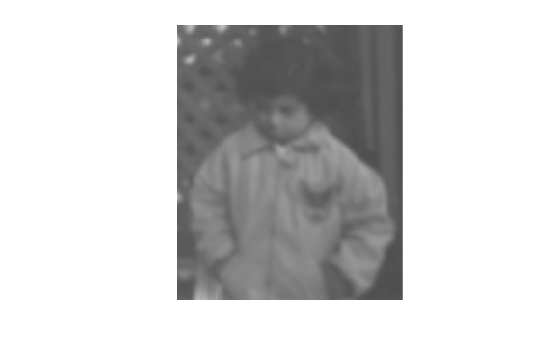Construct Haar-like wavelet filters to find vertical and horizontal edges in an image.

Read the input image and compute the integral image.

```I = imread('pout.tif'); intImage = integralImage(I);```

Construct Haar-like wavelet filters. Use the dot notation to find the vertical filter from the horizontal filter.

```horiH = integralKernel([1 1 4 3; 1 4 4 3],[-1, 1]); vertH = horiH.'```
```vertH = integralKernel with properties: BoundingBoxes: [2x4 double] Weights: [-1 1] Coefficients: [4x6 double] Center: [2 3] Size: [4 6] Orientation: 'upright' ```

Display the horizontal filter.

`imtool(horiH.Coefficients, 'InitialMagnification','fit');`Compute the filter responses.

```horiResponse = integralFilter(intImage,horiH); vertResponse = integralFilter(intImage,vertH);```

Display the results.

```figure; imshow(horiResponse,[]); title('Horizontal edge responses');```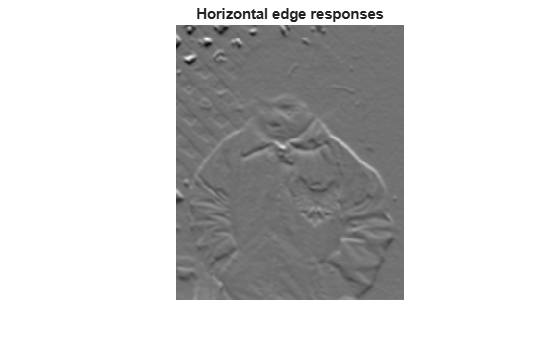```figure; imshow(vertResponse,[]); title('Vertical edge responses');```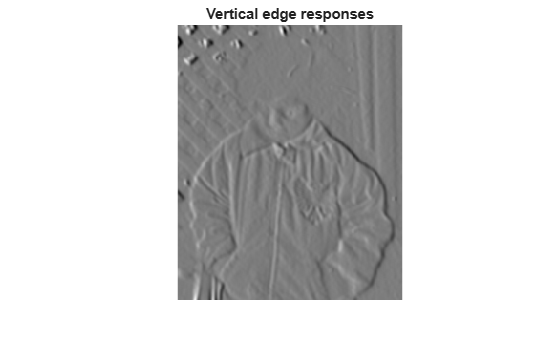`I = imread('pout.tif');`

Compute 45 degree edge responses of the image.

```intImage = integralImage(I,'rotated'); figure; imshow(I); title('Original Image');```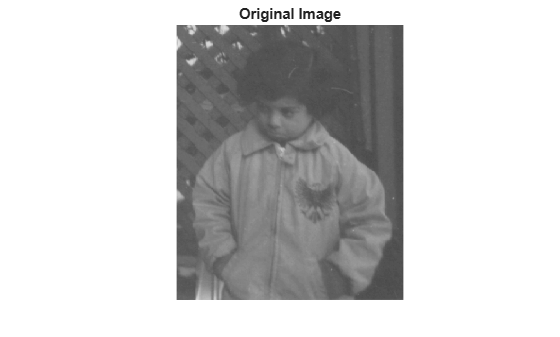Construct 45 degree rotated Haar-like wavelet filters.

```rotH = integralKernel([2 1 2 2;4 3 2 2],[1 -1],'rotated'); rotHTrans = rotH.';```

Visualize the filter rotH.

```figure; imshow(rotH.Coefficients, [],'InitialMagnification','fit');```Compute filter responses.

```rotHResponse = integralFilter(intImage,rotH); rotHTransResponse = integralFilter(intImage,rotHTrans);```

Display results.

```figure; imshow(rotHResponse, []); title('Response for SouthWest-NorthEast edges');```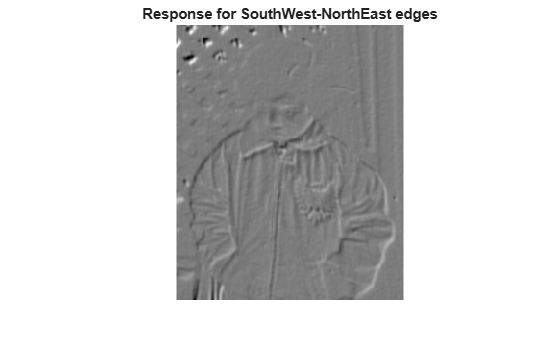```figure; imshow(rotHTransResponse, []); title('Response for NorthWest-SouthEast edges');```Viola, Paul and Michael J. Jones, “Rapid Object Detection using a Boosted Cascade of Simple Features”, Proceedings of the 2001 IEEE Computer Society Conference on Computer Vision and Pattern Recognition, 2001. Volume: 1, pp.511–518.